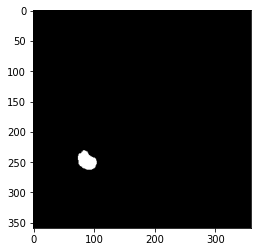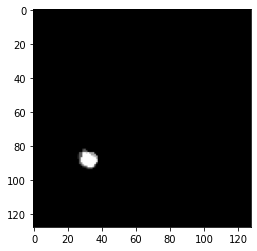I am working with 3D CT images (Dicoms), and I performed several pre-processing steps on the input images such as resampling, normalizing, removing noise, crop image, resizing, crop center and reshaping to (128, 128, 128). I have constructed the 3D segmentation masks (saved as a 3D numpy image) with shape same as the original input image (512,512, x), where x is the number of slices that varies from image to image. How should I pre-process the segmentation mask so that it corresponds iwth the processed input image? Should I register input image and mask? Is there a simple way to do this in sitk?

Hello @DushiFdo,

Possibly consider using TorchIO, highly recommended.

If you want to implement your own, then all you need to do is apply the same spatial transformations to the segmentation mask (remember that when doing resampling you will want to use the `sitk.sitkNearestNeighbor` interpolator). See this Jupyter notebook, function titled `augment_images_spatial` and the parameter of interest is `additional_image_information`.

Thank you. I am not familiar with pytorch so will have to implement my own. The masks I created are numpy arrays, so I guess it won’t have the same information as an Dicom image. So, I am just wondering how I should I proceed in this case?

Hello @DushiFdo,

Assuming you have a CT/MR as a SimpleITK image, let us call it `image` and you have a corresponding mask as a numpy array, let us call it `arr`:

``````mask = sitk.GetImageFromArray(arr)
#Confirm that image and mask are aligned, save both to mha files and
#open in ITK-SNAP

# Use same set of spatial operations on the mask as applied to the image,
# with the only difference being that we use sitkNearestNeighbor when
``````
2 Likes

Thank you so much @zivy. This worked. When resizing the segmentation mask into (128,128,128) after resampling, I use the following code:

``````def resize_volume_mask(img):
"""Resize across z-axis"""
# Set the desired depth
desired_depth = 128
desired_width = 128
desired_height = 128
# Get current depth
current_depth = img.shape #-1
current_width = img.shape #0
current_height = img.shape #1
# Compute depth factor
depth = current_depth / desired_depth
width = current_width / desired_width
height = current_height / desired_height
depth_factor = 1 / depth
width_factor = 1 / width
height_factor = 1 / height
# Rotate
#img = ndimage.rotate(img, 90, reshape=False)
# Resize across z-axis
img = ndimage.zoom(img, (depth_factor, width_factor, height_factor), order=1)
return img
``````

This generates a blurry image with a little different shape for segmentation mask and now the array has values not only 0 and 1, but a series of values between 0 and 1.

The segmentation mask looks as follows, before and after resizing it:
Before:After:I tried the following as well, but the result was same as before.

``````from skimage.transform import resize
image_resized_seg= resize(center_seg, (128, 128, 128), order=1)
``````

Is there a way in sitk to resize the binary segmentation mask, without distorting it?

Hello @DushiFdo,

You are using skimage, so this question should have been posted to the skimage discourse, not here.

Having said that, you need to change to `order=0`, nearest neighbor interpolation, maintaining the original values. `order=1` is linear interpolation so the result includes values that did not exist in the original data.

Thank you. I tried with `order=0` but this removed some slices containing segmentation mask when resizing. That’s why I’d like to do this on `sitk` instead of `skimage`. Is there a function to resize images in `sitk` (for example, from (564, 359, 359) to (128, 128, 128) without loss of information? Here the axis order is (z, x, y).

Hello @DushiFdo,

Yes, this can be done. Please see the data augmentation notebook. The section titled Create reference domain, first code cell. After computing the reference image data you can just use that and call the `Resample` function using the nearest neighbor interpolator.

Hi @zivy, I followed the notebook you linked and created the reference image and then resampled as follows:

``````data = sitk.GetImageFromArray(mask_npa)
dimension = data.GetDimension()

reference_spacing = np.ones(dimension)
reference_origin = np.zeros(dimension)
reference_direction = np.identity(dimension).flatten()
reference_size =  * dimension

reference_image = sitk.Image(reference_size, data.GetPixelIDValue())
reference_image.SetOrigin(reference_origin)
reference_image.SetSpacing(reference_spacing)
reference_image.SetDirection(reference_direction)
reference_center = np.array(
reference_image.TransformContinuousIndexToPhysicalPoint(np.array(reference_image.GetSize()) / 2.0))

resampled_img = sitk.Resample(data, reference_image, sitk.Transform(), sitk.sitkNearestNeighbor, 0.0)
``````

However, this just created an empty image (full black).

Attributes of both mask and reference image are as follows:

``````direction (1.0, 0.0, 0.0, 0.0, 1.0, 0.0, 0.0, 0.0, 1.0)
origin (0.0, 0.0, 0.0)
spacing (1.0, 1.0, 1.0)
``````

Please let me know what needs to be changed. Thank You.

Can someone please tell me what could be the reason for getting a full black image after resampling on to a reference image? I am basically trying to resize a segmentation map.